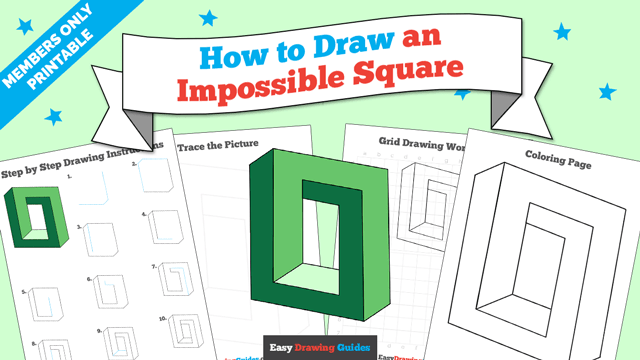# How to Draw an Impossible Square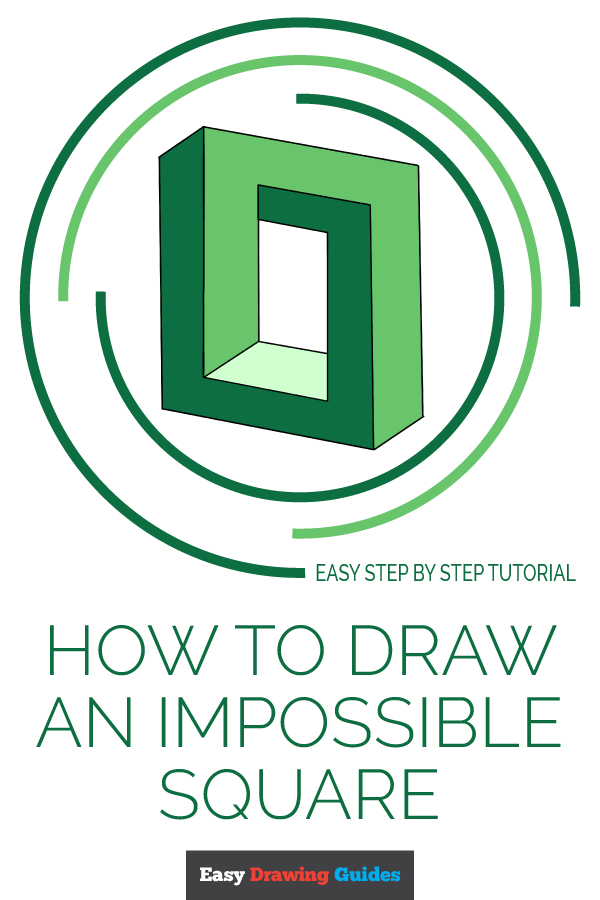The impossible square is a type of impossible object, also known as an impossible figure or undecidable figure. It is a type of optical illusion in which a three-dimensional drawing is interpreted by the eyes and brain as a three-dimensional object. However, its geometry is such that it could not actually exist in three-dimensional space.

The impossible square in this drawing guide is based on the Penrose triangle, an impossible object created by Swedish artist Oscar Reutersvard in 1934. Therefore, it is sometimes called a Penrose Square.

Notice the angles at which the sides of the square seem to be oriented. Then, notice how these angles appear to connect. The impossibility of the figure confuses the mind and has made it a popular art object for nearly a century.

Optical illusions such as the impossible square use shading to help trick the brain. Notice the darker and lighter shading that appears to indicate shadows. Our previous knowledge of angles also adds to the illusion. Even once the impossibility of the object is realized, the seemingly three-dimensional nature remains.

Interestingly, other Penrose or impossible shapes can be made, including pentagons, hexagons, and octagons. However, as the number of sides increases, the optical illusion is less striking. Rather than appearing "impossible," the figures seem to be warped, twisted, or braided.

Would you like to draw an impossible square? This easy, step-by-step shape drawing tutorial is here to help. All you will need is a piece of paper and a pencil, marker, or pen. You may also wish to color your finished drawing.

If you liked this tutorial, see also the following drawing guides: Impossible Triangle, Impossible Cube, and Star.

## ​Step by Step Instructions for Drawing​ an Impossible Square1. Begin by drawing a straight vertical line. This indicates one side of the square. From the bottom of this line, extend a short, straight, diagonal line. Then, from the end of this line, extend a longer straight line on the opposite diagonal. These lines form the corner and bottom side of the square.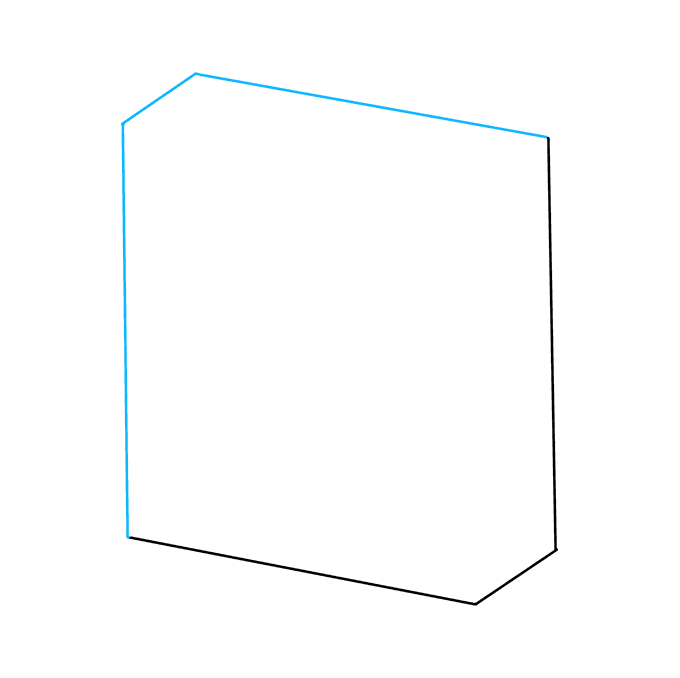2. Extend a long, straight, vertical line upward from the bottom of the square. From this line, extend a shorter, straight diagonal line. Then, connect this to the original line using a long straight line.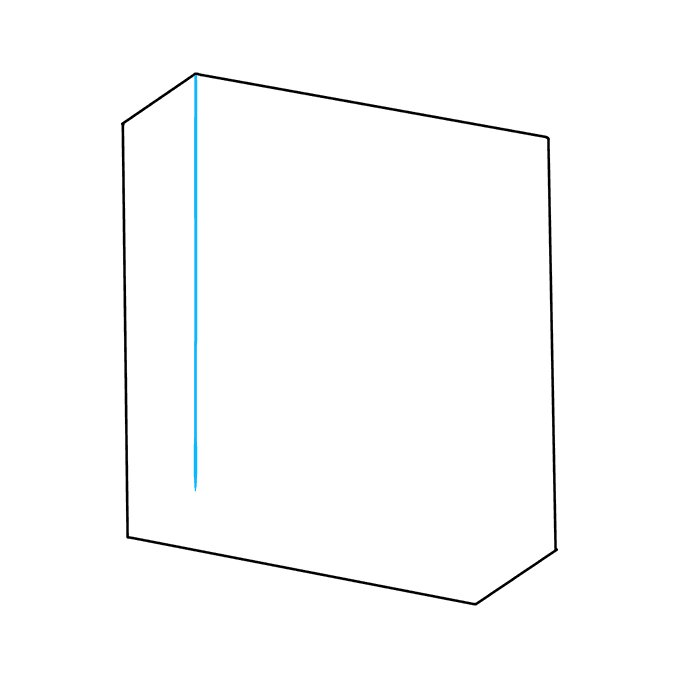3. Draw a straight vertical line downward from the uppermost corner of the figure. Do not extend this line as far as the bottom of the square.4. Draw a straight diagonal line extending from the previous line. This line should be parallel to the bottom of the square, outlining the top of the bottom segment.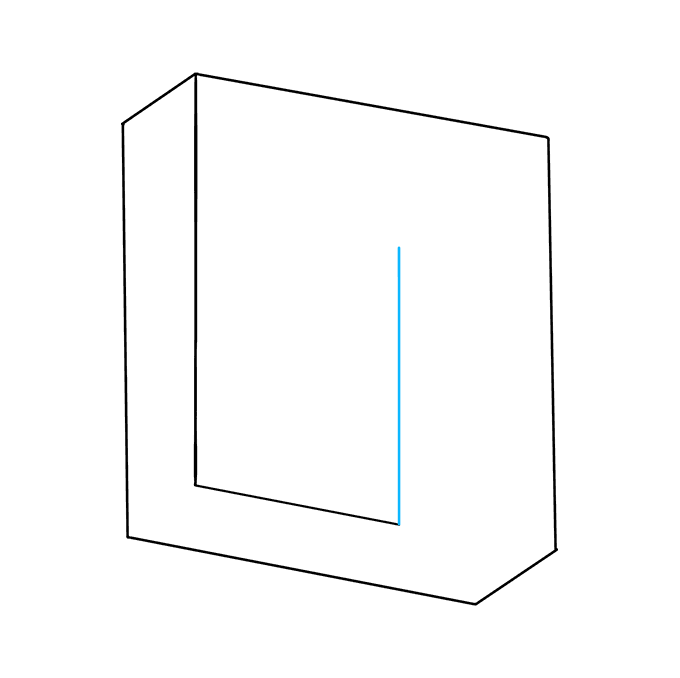5. Draw a straight line upwards from the end of the previous line. This line should be parallel to the side of the square, but notice that it is farther away from the exterior line than the previous lines have been.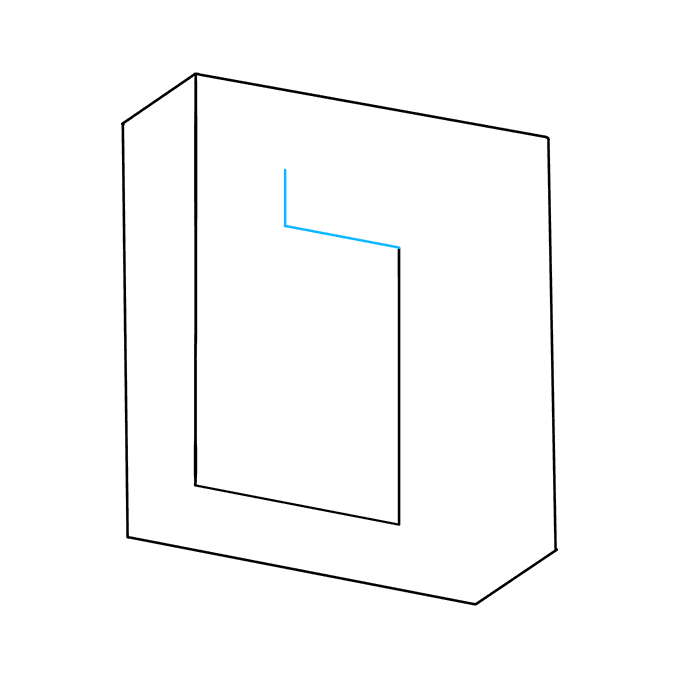6. From the end of the previous line, draw a short straight line parallel to the top of the square. Then, draw a shorter straight line upward from the end of this line.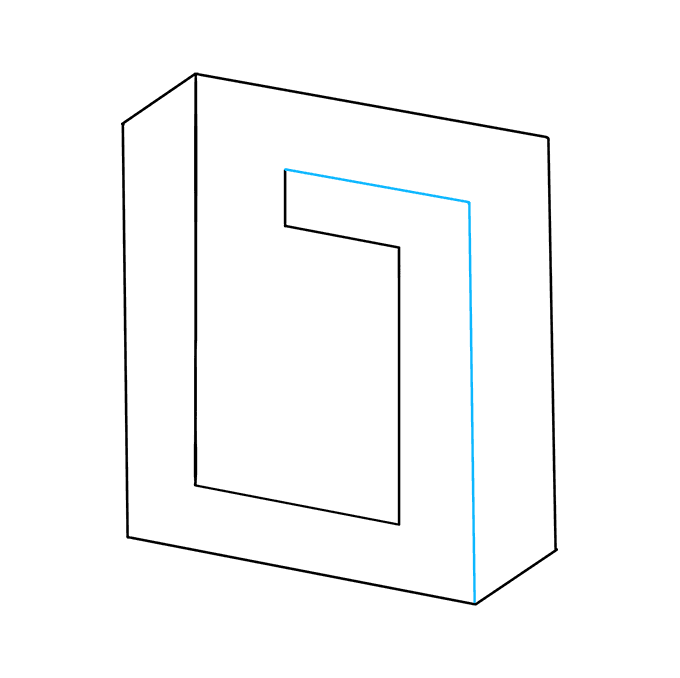7. From the end of the previous line, draw a straight line parallel to the top of the square. Then, draw a straight line downward to meet the bottommost corner of the square.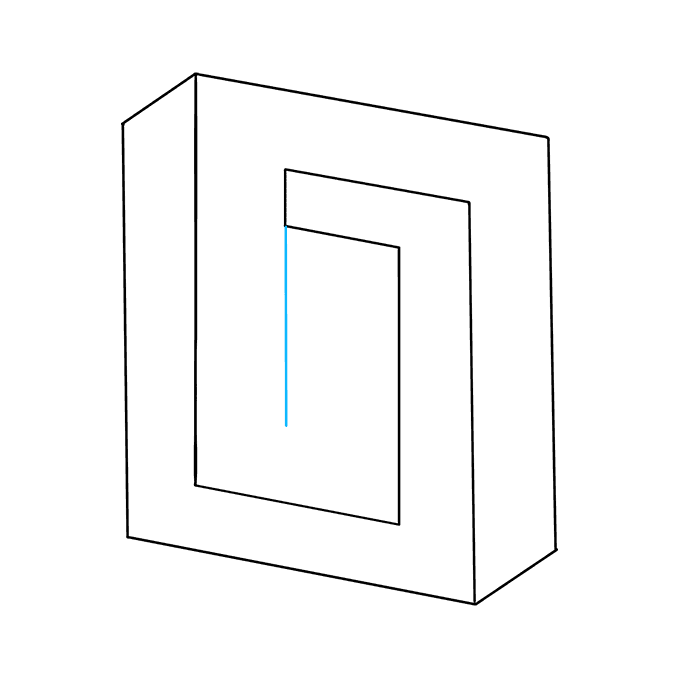8. Next, you will finish outlining the interior of the square. From the inner corner of the shape enclosed in the previous step, draw a straight vertical line.9. From the previous line, draw a straight line to meet the opposite edge of the square's interior. Connect the interior corner with the next closest corner to complete the impossible shape.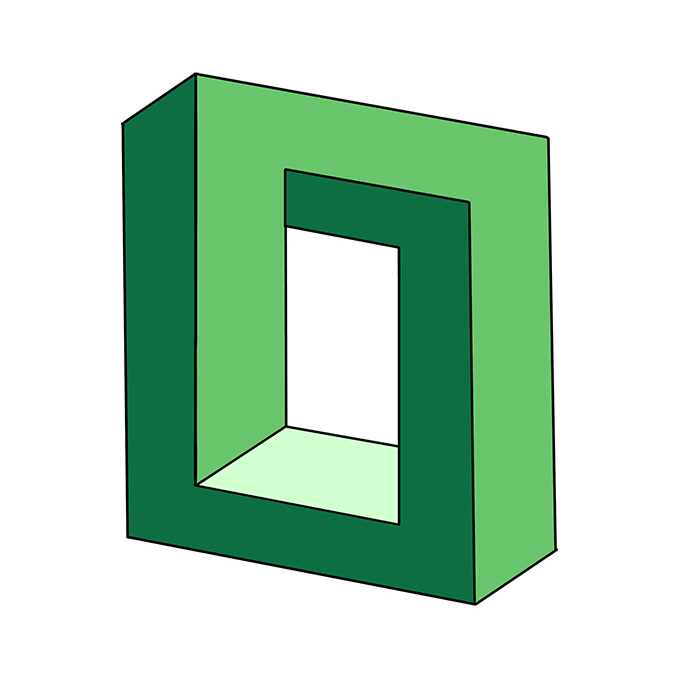10. Color your impossible square. Then, check out our man-made objects drawing guides to learn to draw more impossible shapes.

## The Complete Impossible Square Drawing Tutorial in One Image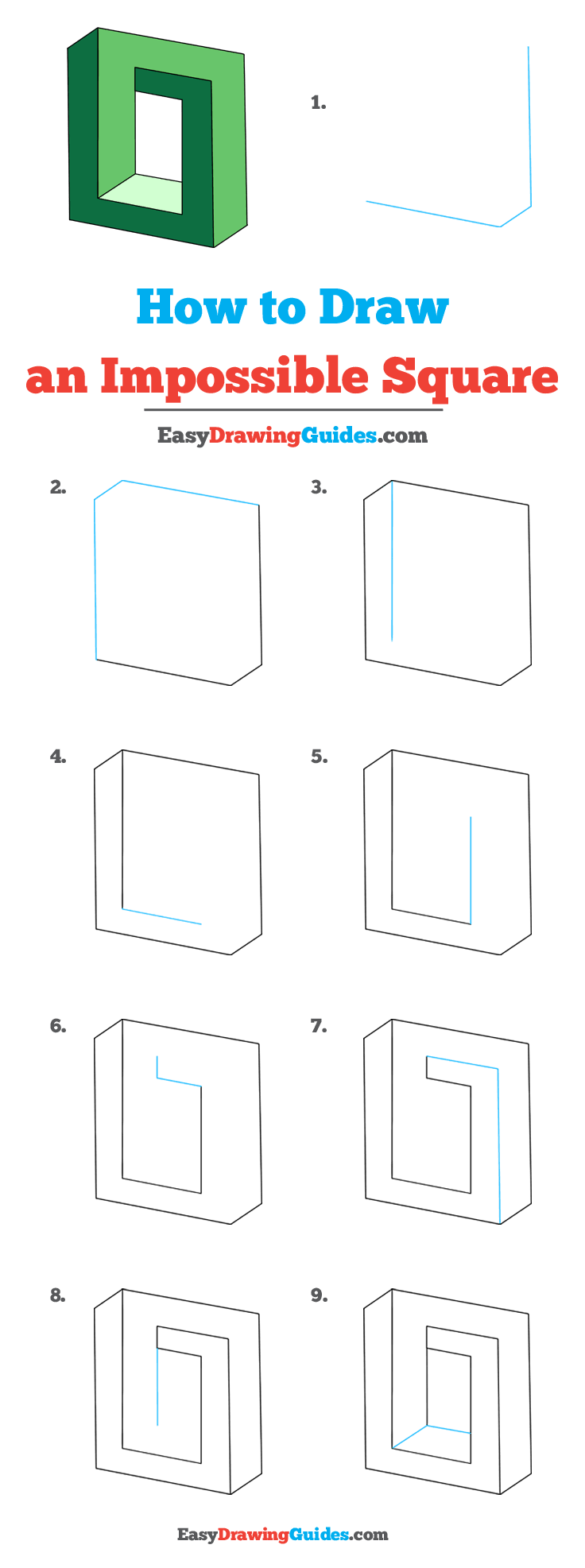## Printable Drawing Tutorial

This printable is for members only.